\n"; } \$col_width = 100 / \$col_count; print "\n"; if(\$i % \$col_count == \$col_count) { print "\n"; } } } else if(\$_REQUEST["v"] == "l") { /* List View */ print "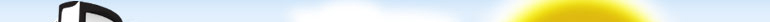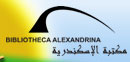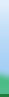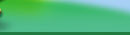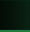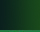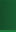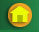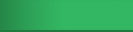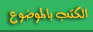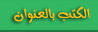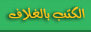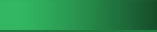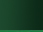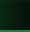\n"; print _('Collection') . ": " . \$_REQUEST["c"] . "" . "\n"; print ""; } ?>
 " . \$collection_count . "" . "\n"; } else { print _('Book Count') . ": " . \$book_count . "" . "\n"; } ?>
\n"; \$col_count = 3; for(\$i = 1; \$book = mysqli_fetch_array(\$result, MYSQLI_ASSOC); \$i++) { if(\$i % \$col_count == 1) { print "
\n"; print "\n"; printf("\n", "book.php?b=" . \$book["bdn"], \$book["title"], "books/" . \$book["bdn"] . "_COVER_s.jpg"); /* printf("\n", \$book["title"]); */ print "%s
\n"; print "
\n"; print "\n"; for(\$i = 1; \$book = mysqli_fetch_array(\$result, MYSQLI_ASSOC); \$i++) { if(\$i % 2 == 1) { print "\n"; } else { print "\n"; } printf("\n", "book.php?b=" . \$book["bdn"], \$book["title"], \$book["author"], \$book["year"], \$book["page_count"]); print "\n"; } } else if(\$_REQUEST["v"] == "t") { /* Theme View */ print "
 " . _('Title') . " " . _('Author') . " " . _('Publication Date') . " " . _('Page Count') . " %s %s %s %s
\n"; \$col_count = 3; for(\$i = 1; \$book = mysqli_fetch_array(\$result, MYSQLI_ASSOC); \$i++) { if(\$i % \$col_count == 1) { print "\n"; } \$col_width = 100 / \$col_count; print "\n"; if(\$i % \$col_count == \$col_count) { print "\n"; } } } mysqli_close(\$con); ?>
\n"; print "\n"; printf("\n", "browse.php?v=c&c=" . \$book["subject"] . " ", \$book["subject"]); printf("\n", "browse.php?v=c&c=" . \$book["subject"] . " ", \$book["title"], "books/" . \$book["bdn"] . "_COVER_s.jpg"); /* printf("\n", \$book["title"]); */ print "
 %s%s
\n"; print "